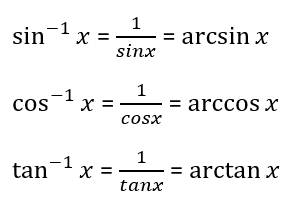# Inverse Trig Functions Worksheets

What are Inverse Trig Functions? In the world trigonometry, some functions are extensively used for a higher level of problems, i.e., the inverse trigonometric functions. You are aware of the trigonometric functions (sin, cos, and tan), which is used to find the unknown side length of a right triangle. The inverse trigonometric functions sin-1, cos-1, and tan-1 are used to find the unknown measure of the angle of a right-angled triangle when you are of the two side lengths. In other words, the inverse trigonometric functions sin-1, cos-1, and tan-1 can be denoted as 1/sin, 1/cos, and 1/tan respectively.• ### Basic Lesson

Guides students solving equations that involve an Inverse Trig Functions. Demonstrates answer checking. Find the value of Θ in radians considering the principal inverse function: Θ = arc sin(√3/2). The inverse function arcsin(x)= sin-1(x)and arcsin can be read as "the angle whose sine is". According to the question it means the angle whose sin is √3/2.

• ### Intermediate Lesson

Demonstrates how to solve more difficult problems. In a right triangle the legs have lengths 4 and 5. Find the expression for angle B?

• ### Independent Practice 1

A really great activity for allowing students to understand the concept of Inverse Trig Functions.

• ### Independent Practice 2

Students find the Inverse Trig Functions in assorted problems. The answers can be found below.

• ### Homework Worksheet

Students are provided with problems to achieve the concepts of Inverse Trig Functions.

• ### Skill Quiz

This tests the students ability to evaluate Inverse Trig Functions.